# 利用OpenCV实现软件自动识别核酸检测时间

### 文章目录

• 问题介绍
• 一、前期准备工作
• 1.Python的安装
• 2.安装OpenCV-Python开发包
• 3.安装Tesseract-OCR
• 二、编写程序
• 1.引入库
• 2.使用Tesseract做文字识别（OCR）
• 3.筛选日期
• 4.日期运算
• 5.运行程序
• 总结

• # 问题介绍

`在程序工程训练课程中，出现了一道题：`

手机截屏核酸检测报告，利用OpenCV实现软件自动识别核酸检测时间是否在72小时之内。

# 一、前期准备工作

实现本任务需要的软件：

``````- Python3.x
- OpenCV-Python 4.x
- Tesseract-OCR 5.x
- Win10 64 或 Win11 64
``````

## 1.Python的安装

安装Python SDK时选择默认安装，同时勾选将其添加到环境变量中。
闲麻烦的可以直接从Microsoft Store下载
或者安装PyCharm
PyCharm下载地址

## 2.安装OpenCV-Python开发包

调用cmd，或在终端中使用pip指令安装：

``````pip install opencv-python
``````

如果下载网速较慢，可以使用清华镜像源地址，需要在命令结尾加上-i https://pypi.tuna.tsinghua.edu.cn/simple/
完整指令：

``````pip install opencv-python -i https://pypi.tuna.tsinghua.edu.cn/simple/
``````

## 3.安装Tesseract-OCR

官方网站
官方文档
语言包地址
下载地址
首先安装Tesseract-OCR Python SDK支持，调用cmd，或在终端中使用pip指令安装：

``````pip install pytesseract -i https://pypi.tuna.tsinghua.edu.cn/simple/
``````

然后点击下载地址链接安装Tesseract-OCR最新版本，然后在环境变量中添加：

``````C:\Program Files\Tesseract-OCR
``````

安装与配置好OpenCV-Python与Tesseract-OCR之后，需要进一步通过代码验证正确性。打开Pycharm IDE，新建一个python项目与python文件，输入以下代码：

``````import pytesseract as tess
print(tess.get_tesseract_version())
print(tess.get_languages())
``````

或者在终端中输入：

``````tesseract -v
``````

如果安装成功，会显示对应版本号和适用语言。
最后点击语言包地址链接安装chi_sim中文简体语言包，下载后将该包直接放在程序安装目录的tessdata文件夹里面即可。
命令行使用 tesseract –list-langs命令可查看当前软件支持的语言：

``````tesseract --list-langs
``````

# 二、编写程序

## 1.引入库

本程序需要的库函数，代码如下（示例）：

``````import cv2 as cv
import pytesseract as tess
from datetime import datetime
``````

## 2.使用Tesseract做文字识别（OCR）

OCR（optical character recognition，光学字符识别）是指直接将包含文本的图像识别为计算机文字（计算机黑白点阵）的技术。图像中的文本一般为印刷体文本。
Tesseract是github上的OCR开源库，今天我们将使用Tesseract来进行文字识别。
比如我们需要识别核酸检测报告上的文字：输入以下代码进行测试：

``````import cv2 as cv
import pytesseract as tess
image_rgb = cv.cvtColor(image, cv.COLOR_BGR2RGB)
text = tess.image_to_string(image_rgb, lang="eng")
content = text.replace("\f", "").split("\n")
txt = []
for c in content:
if len(c) > 0:
print(c)
h, w, c = image.shape
boxes = tess.image_to_boxes(image)
for b in boxes.splitlines():
b = b.split(' ')
image = cv.rectangle(image, (int(b), h - int(b)), (int(b), h - int(b)), (0, 255, 0), 2)
cv.imshow('text detect', image)
cv.waitKey(0)
cv.destroyAllWindows()
``````

运行结果：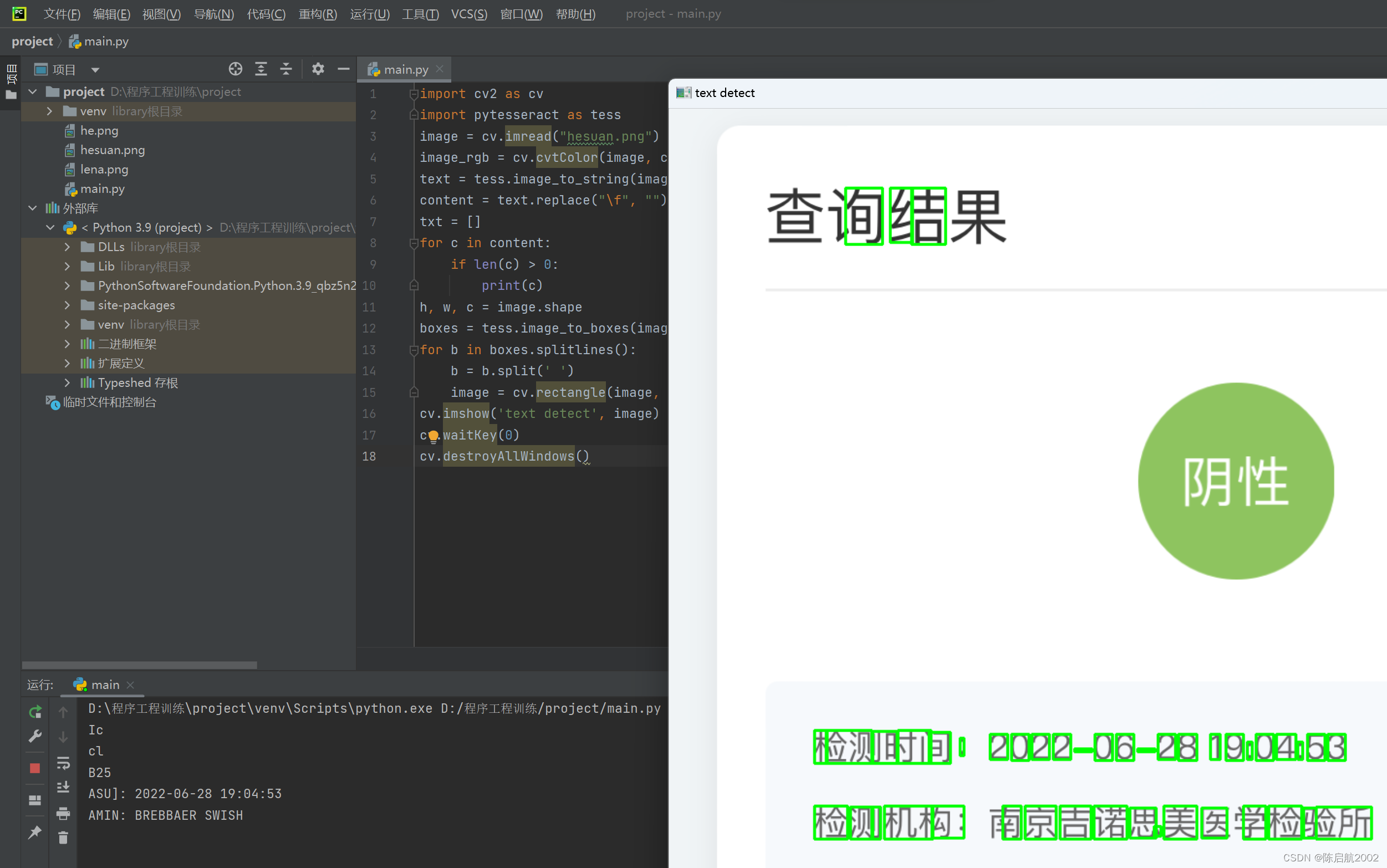可以看到图片中的信息被很好地识别了出来。
但是在输出中出现了乱码：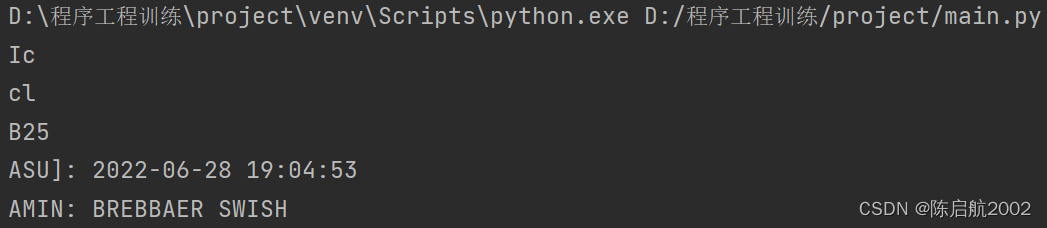是因为我们选用的是英文标准库，如果需要识别中文我们需要将代码中lang的参数改为中文简体：

``````text = tess.image_to_string(image_rgb, lang="chi_sim")
``````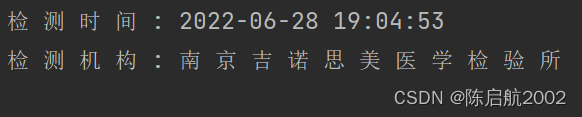中文和对应的日期成功识别。

## 3.筛选日期

利用列表和字符串遍历可以快速筛选出日期信息。

``````for c in content:
if len(c) > 0:
txt.append(c)
for i in txt:
if i=='检' and i=='测' and i=='时' and i=='间':
ret=i
break
else:
ret=False
print(ret)
time = ret[10:]
print(time)
``````

可以得到对应结果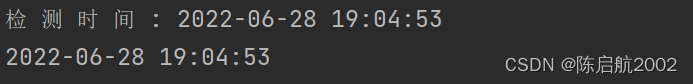## 4.日期运算

Python 中的日期不是其自身的数据类型，但是我们可以导入名为 datetime 的模块，把日期视作日期对象进行处理。
datetime是模块，datetime模块还包含一个datetime类，通过from datetime import datetime导入的才是datetime这个类。

``````from datetime import datetime
``````

用户输入的日期和时间是字符串，要处理日期和时间，首先必须把str转换为datetime。转换方法是通过datetime.strptime()实现，需要一个日期和时间的格式化字符串：

``````date = datetime.strptime(time, '%Y-%m-%d %H:%M:%S')
``````

使用datetime.now()可以获取系统日期：

``````print(datetime.now())
``````

格式化后的日期可以作减法，并通过delta.days()函数显示对应天数。

``````delta = datetime.now() - date
print(delta.days)
if(delta.days<=3):
print("核酸报告在三天之内")
else:
print("核酸报告不在三天之内")
``````

## 5.运行程序

运行程序，得到相应结果。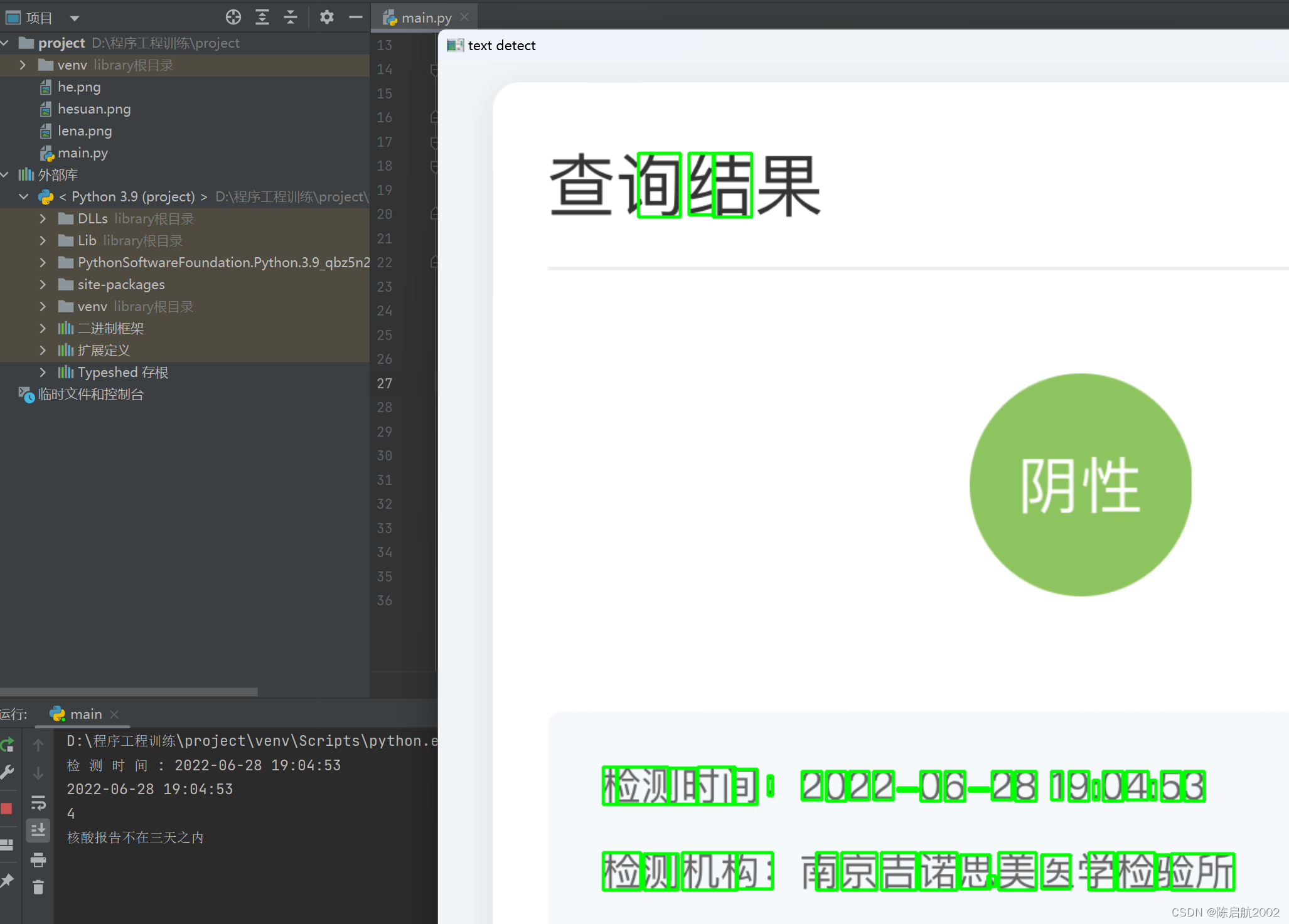# 总结

本文介绍了利用OCR方案，基于OpenCV实现软件自动识别核酸检测时间是否在72小时之内。程序能够准确运行的前提是截图清晰可见，如果用拍摄的图像作文字识别，需要利用OpenCV进行更多的图像处理，如二值化、投影变换等。

来源：陈启航2002